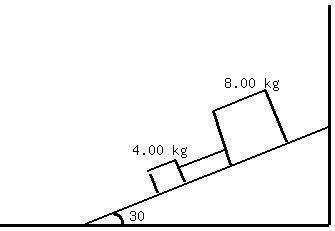# Two blocks with masses 4.00 kg and 8.00 kg are connected by string and slide down a 30.0 degree inclined plane.foass77W 2020-12-28 Answered

Two blocks with masses 4.00 kg and 8.00 kg are connected by string and slide down a 30.0 degree inclined plane. The coefficient of kinetic friction between the 4.00-kg block and the plane is 0.25; that between the 8.00-kg block and the plane is 0.35.
a) Calculate the acceleration of each block
b) Calculate the tension in the string
c) What happens if the positions of the block are reversed, so that the 4.00-kg block is above the 8.00-kg block?You can still ask an expert for help

• Questions are typically answered in as fast as 30 minutes

Solve your problem for the price of one coffee

• Math expert for every subject
• Pay only if we can solve ithajavaF
First note that you must establish a reference frame (i.n. xy plane). For convenience, it is generally assumed that thex-axis is parallel to the slope of the surface and the y-axis is perpendicular to the slope. Furthermore, the positive x-direction is down slope.
Given the above assumptions, now determine the sum of the forces for each block.
${m}_{1}$=4kg mass of block 1
${m}_{2}$=8kg mass of block 2
${u}_{1}$=0.25 coefficient of friction for block 1
${u}_{2}=0.35$ coefficient of friction for block 2
slope of ramp
g = acceleration due to gravity = 9.8m/s
sum of forces on block 1:
Note that the coefficient of friction is applied to the force normal to the surface
$\sum {F}_{1}={m}_{1}\cdot g\mathrm{sin}\left(\alpha \right)-{u}_{1}\cdot {m}_{1}\cdot g\cdot \mathrm{cos}\left(\alpha \right)=11.1129$ newtons
where ${m}_{1}\cdot g\cdot \mathrm{sin}\left(\alpha \right)$ is the force in the positive x-directionon block 1 due to its weight and ${m}_{1}\cdot g\cdot \mathrm{cos}\left(\alpha \right)$ is the normal force, perpendicular to the slope, due to acceleration of gravity.
Given the sum of forces for F1, its
###### Not exactly what you’re looking for?Jeffrey Jordon

${n}_{1}={m}_{1}g\mathrm{cos}30$

${f}_{k1}={\mu }_{k1}{m}_{1}g\mathrm{cos}30$

${m}_{1}{a}_{1}={m}_{1}g\mathrm{sin}30-{\mu }_{k1}{m}_{1}g\mathrm{cos}30$

${a}_{1}=9.8\left(\mathrm{sin}30-0.2\mathrm{cos}30\right)=3.20\frac{m}{{s}^{2}}$

${n}_{2}={m}_{2}g\mathrm{cos}30$

${f}_{k2}={\mu }_{k2}{m}_{2}g\mathrm{cos}30$

${m}_{2}{a}_{2}={m}_{2}g\mathrm{sin}30-{\mu }_{k2}{m}_{2}g\mathrm{cos}30$

${a}_{2}=9.8\left(\mathrm{sin}30-0.4\mathrm{cos}30\right)=1.51\frac{m}{{s}^{2}}$

b)

${m}_{1}{a}_{1}={m}_{1}g\mathrm{sin}30-{\mu }_{k1}{m}_{1}g\mathrm{cos}30-T$

${m}_{2}{a}_{2}={m}_{2}g\mathrm{sin}30-{\mu }_{k2}{m}_{2}g\mathrm{cos}30+T$

$T=\frac{4\cdot 8\left(3.2-1.51\right)}{4+8}=4.51N$